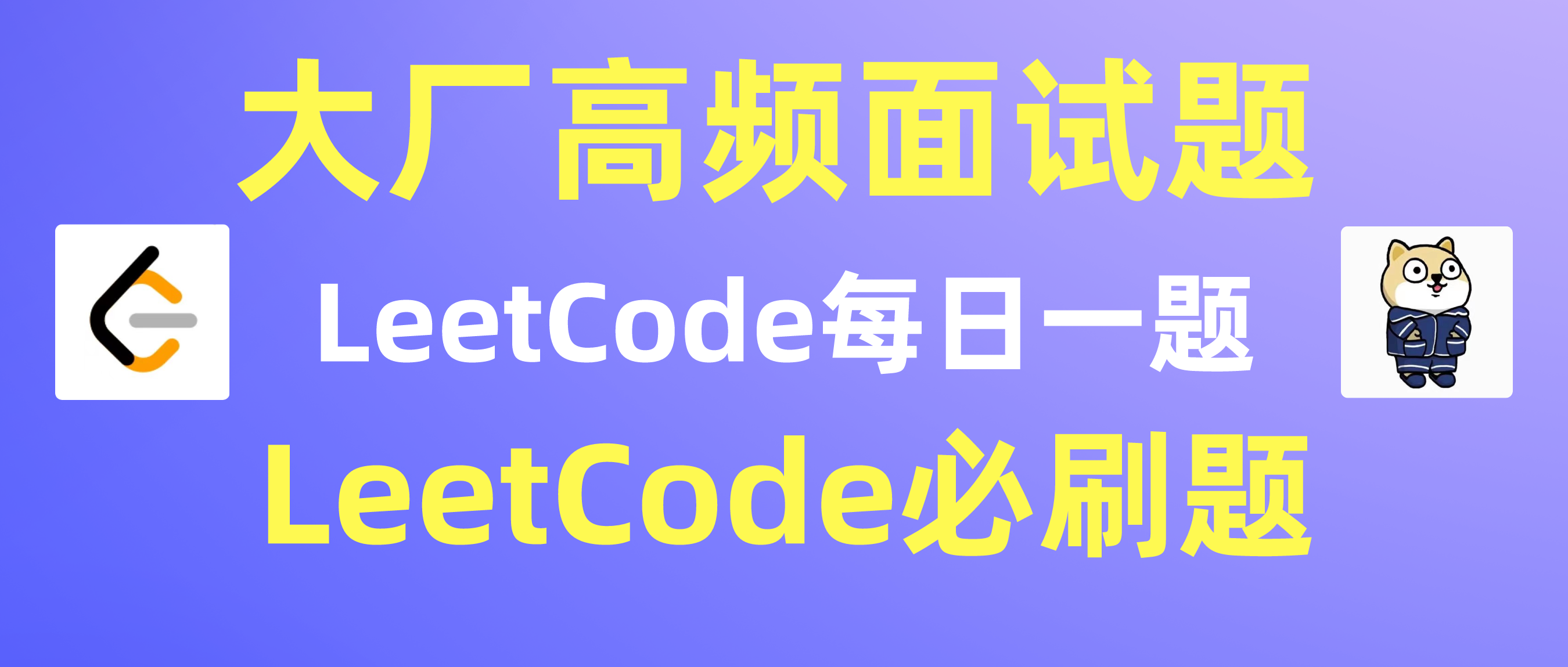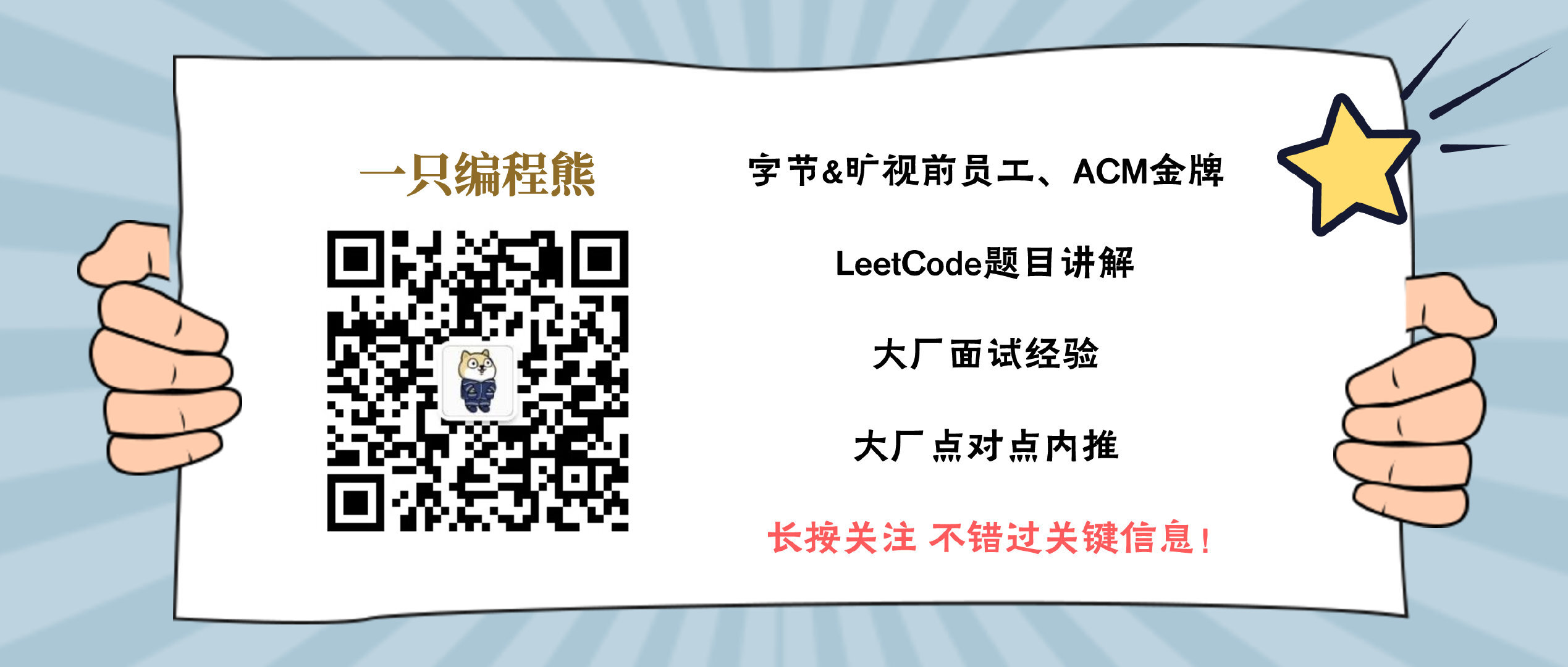【LeetCode 每日一题 Day 3】3. 无重复字符的最长子串题解

C++代码

class Solution {public:    queue<char> q;    int lengthOfLongestSubstring(string s) {        vector<char> cnt(128, 0);        int ans = 0;        for (int i = 0; i < int(s.size()); i++) {            q.push(s[i]), cnt[s[i]]++;            while (cnt[s[i]] > 1) {                char frontC = q.front();                q.pop();                cnt[frontC]--;            }            ans = max(ans, int(q.size()));        }        return ans;    }};

Java 代码

class Solution {    public int lengthOfLongestSubstring(String s) {        char[] cnt = new char;        LinkedList<Character> q = new LinkedList<Character>();        int ans = 0;        for (int i = 0; i < s.length(); i++) {            q.add(s.charAt(i));            cnt[s.charAt(i)]++;            while (cnt[s.charAt(i)] > 1) {                char frontC = q.pollFirst();                cnt[frontC]--;            }            ans = Math.max(ans, q.size());        }        return ans;    }}评论